# KSEEB Solutions for Class 6 Maths Chapter 7 Fractions Ex 7.4

Students can Download Chapter 7 Fractions Ex 7.4 Questions and Answers, Notes Pdf, KSEEB Solutions for Class 6 Maths helps you to revise the complete Karnataka State Board Syllabus and score more marks in your examinations.

## Karnataka State Syllabus Class 6 Maths Chapter 7 Fractions Ex 7.4

Question 1.
Write shaded portion as fraction. Arrange them in ascending and descending order using correct sign ‘<‘, ‘=’, ‘>’ between the fractions:-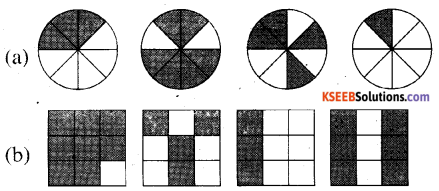c) Show $$\frac{2}{6}$$, $$\frac{4}{6}$$, $$\frac{8}{6}$$ and $$\frac{6}{6}$$ on the number line. Put appopriate signs between the fractions given.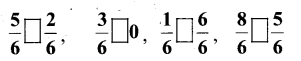a) * Here, 1st circle represents 3 shaded parts out of 8 equal parts. Therefore, it represents a fraction $$\frac{3}{8}$$
* Here, 2nd circle represents 6 shaded parts out of 8 equal parts. Therefore, it represents a fraction $$\frac{6}{8}$$
* Here, 3rd circle represents 4 shaded parts out of 8 equal parts. Therefore, it represents a fraction $$\frac{4}{8}$$
* Here, 4th circle represents 1 shaded parts out of 8 equal parts. Therefore, it represents a fraction $$\frac{1}{8}$$
Now, these fractions may be arranged as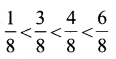b) * Here, 1st square represents 8 shaded parts out of 9 equal parts Therefore, it represents a fraction $$\frac{8}{9}$$
* Here, 2nd square represents 4 shaded parts out of 9 equal parts Therefore, it represents a fraction $$\frac{4}{9}$$
* Here, 3rd square represents 3 shaded parts out of 9 equal parts Therefore, it represents a fraction $$\frac{3}{9}$$
* Here, 4th square represents 6 shaded parts out of 9 equal parts Therefore, it represents a fraction $$\frac{6}{9}$$
Now, these fractions can be arranged as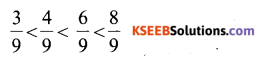c) To represent the given fraction
$$\frac{2}{6}$$, $$\frac{4}{6}$$, $$\frac{8}{6}$$ and $$\frac{6}{6}$$ on number line, each unit length should be succeed in 6 equal parts Now, these fractions can be represented as.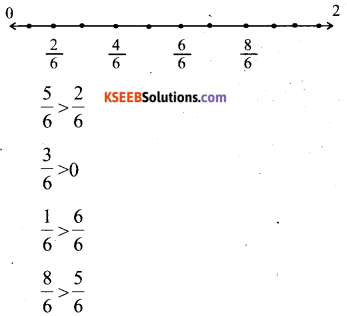Question 2.
Compare the fractions and put an appropriate sign.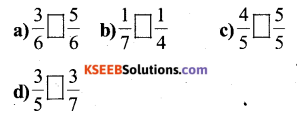Solution: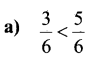Here, the denominator are same, Therefore, The fraction having the greater numerator will be greater.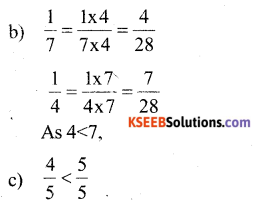Here, the denominators are same, Therefore the fraction having the greater numerator will be greater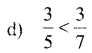Question 3.
Make five more such pairs and put appropriate signs.
Solution: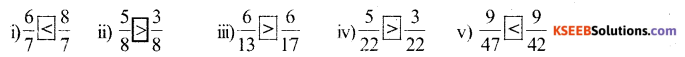Question 4.
Look at the figures and write ‘<’ or ‘>% ‘=’ between the given pairs of fractions.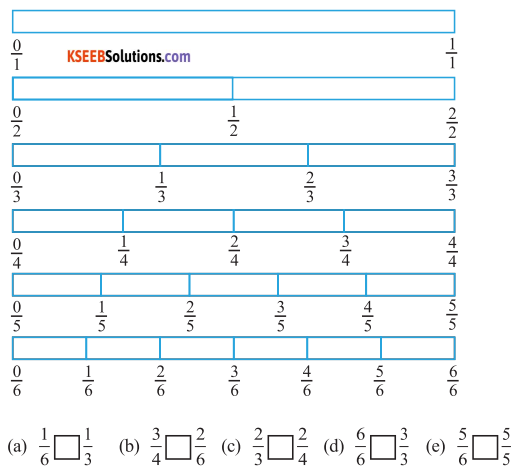Solution: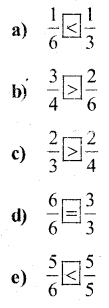Question 5.
How quickly can you do this? Fill appropriate sign( ‘<% ‘=’, ‘>’)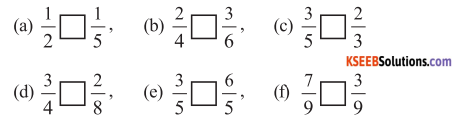Solution: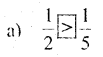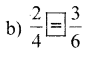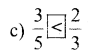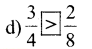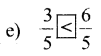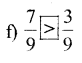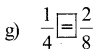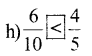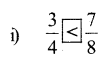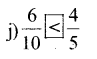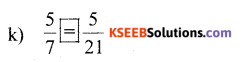Question 6.
The following fractions represent just three different numbers. Separate them into three groups of equivalent fractions, by changing each one to its simplest form.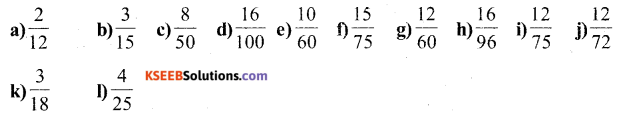Solution: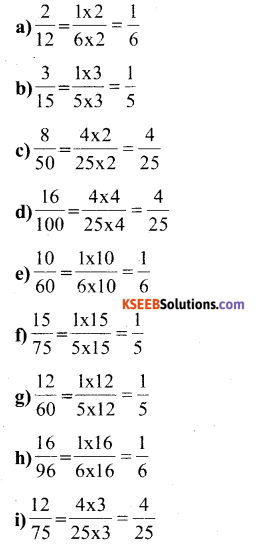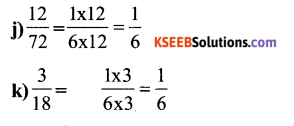l) $$\frac{4}{25}$$ = there are 3 groups of equivalent fractions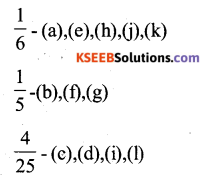Question 7.
Find answers to the following. Write and indicate how you solved them.
Solution:
a) Is $$\frac{5}{9}$$ equal to $$\frac{4}{5}$$?
Converting these into like fractions,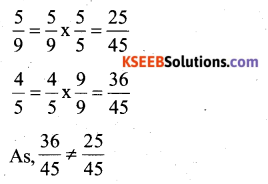Therefore, $$\frac{5}{9}$$ is not equal to $$\frac{4}{5}$$

b) Is $$\frac{9}{16}$$ equal to $$\frac{5}{9}$$ ?
Converting these into like fractions,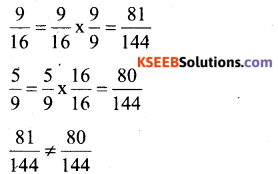Therefore, $$\frac{9}{16}$$ is not equal to $$\frac{5}{9}$$

c) Is $$\frac{4}{5}$$ equal to $$\frac{16}{20}$$?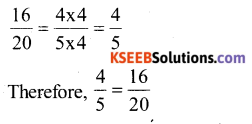d) Is $$\frac{1}{15}$$ equal to $$\frac{4}{30}$$?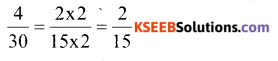Therefore, $$\frac{1}{15}$$ is not equal to $$\frac{4}{30}$$Question 8.
Ila read 25 pages of a book containing 100 pages, Lalita read $$\frac{2}{5}$$ of the same book. Who read less?
Solution:
Numbers of pages read by Lalita =
$$\frac{2}{5} \times 100=40$$
Number of pages read by Ila = 25
Hence, Ila has read less number of pages

Question 9.
Rafiq exercised for $$\frac{3}{6}$$ of an hour, while Rohit exercised for $$\frac{3}{4}$$ of an hour Who exercised for a longer time?
Solution:
Rafiq exercised for $$\frac{3}{6}$$ hr and Rohit exercised for $$\frac{3}{4}$$ hr converting these into like fractions.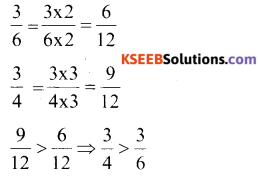Hence, Rohit exercised for a longer time.Question 10.
In a class A of 25 students 20, passed in first class; in another class B of 30 students, 24 passed in first class. In Which class was a greater fraction of students getting first class?
Solution:
Fraction of students of class A who passed in 1st class = $$\frac{20}{25}=\frac{4}{5}$$
Fraction of student of class B Who passed in 1st class = $$\frac{24}{30}=\frac{4}{5}$$
From both classes, an equal fraction of student passed in first class